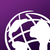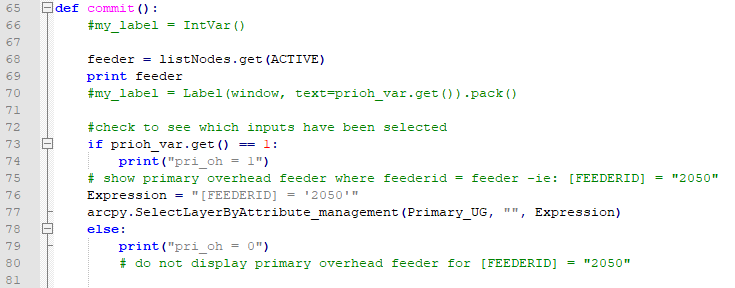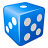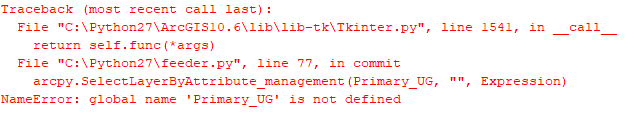# error: global name 'Primary_OH' is not defined

3280
43
12-27-2019 11:14 AMNew Contributor II

I am trying to run my program and when i get to this part of the programI get the error that global name 'Primary_OH is not defined. Can someone look at my code and tell what i need to do to fix this.

Thanks

Tags (1)
1 Solution

Accepted SolutionsbyEsri Contributor

OK. It does not matter. The container of data is a Personal Geodatabase, is so similar to File Geodatabase

```
# Primary OH Path
geodatabase = "C:\gismaps\LusData.mdb"
dataset = "ElectricData"
feature_class = "PriOHElectricLineSegment"
Primary_OH_path = r"{0}\{1}\{2}".format(geodatabase, dataset, feature_class)
Primary_OH = arcpy.MakeFeatureLayer_management(Primary_OH_path, "Primary_OH")

```
43 RepliesbyMVP Esteemed Contributor

Your code would be a lot easier to trouble shoot if you paste it into the syntax highlighter rather than providing it as an image.  I don't see a reference to Primary_OH in the what you display, but I might be missing it. Somewhere in your code you call it and it should be in quotes is my guess...

That should just about do it....New Contributor II

Joe,

Thanks for the response. Line 77 is where the error is

``````from tkinter import *
from tkinter import messagebox
import Tkinter, arcpy

myMap = arcpy.mapping.MapDocument("C:/GISMAPS/LUS Map.mxd")
#myMap = arcpy.mapping.MapDocument("CURRENT")

window = Tk()
window.title("Feeders Selection")
window.geometry("680x500")
window.iconbitmap('C:/GISMAPS/lus.ico')

#Define the frame for the feeders to be displayed
frame = Frame(window)
listNodes = Listbox(frame, width=10, height=10, font=("Helvetica", 10))
listNodes.pack(side="left", fill="y")

#Define the scrollbar in frame window
scrollbar = Scrollbar(frame, orient="vertical")
scrollbar.config(command=listNodes.yview)
scrollbar.pack(side="right", fill="y")
listNodes.config(yscrollcommand=scrollbar.set)

#Add the selection of all feeders
for item in [2050, 2051, 2052,
3050, 3051, 3052, 3550,
4050, 4051, 4052,
5050, 5051, 5052]:
listNodes.insert(END, item)
feeder = listNodes.get(ACTIVE)

#Define all of the checkboxes
prioh_var = IntVar()
priug_var = IntVar()
secoh_var = IntVar()
secug_var = IntVar()
fuse_var = IntVar()
switch_var = IntVar()
sp_var = IntVar()
cap_var = IntVar()
rec_var = IntVar()
pinhook_var = IntVar()
mall_var = IntVar()
peck_var = IntVar()
pri_oh = Checkbutton(window, text = "Primary Overhead", variable = prioh_var,onvalue = 1, offvalue = 0, height=2, width = 20).place(x=2, y=175)
pri_ug = Checkbutton(window, text = "Primary Underground", variable = priug_var,onvalue = 1, offvalue = 0, height=2, width = 20).place(x=12, y=200)
sec_oh = Checkbutton(window, text = "Secondary Overhead", variable = secoh_var,onvalue = 1, offvalue = 0, height=2, width = 20).place(x=8, y=225)
sec_ug = Checkbutton(window, text = "Secondary Underground", variable = secug_var,onvalue = 1, offvalue = 0, height=2, width = 20).place(x=18, y=250)
fuse = Checkbutton(window, text = "Fuse", variable = fuse_var,onvalue = 1, offvalue = 0, height=2, width = 20).place(x=400, y=10)
switch = Checkbutton(window, text = "Switch", variable = switch_var,onvalue = 1, offvalue = 0, height=2, width = 20).place(x=405, y=35)
servicepoint = Checkbutton(window, text = "Service Point", variable = sp_var,onvalue = 1, offvalue = 0, height=2, width = 20).place(x=420, y=88)
capacitor = Checkbutton(window, text = "Capacitor", variable = cap_var,onvalue = 1, offvalue = 0, height=2, width = 20).place(x=412, y=60)
recloser = Checkbutton(window, text = "Recloser", variable = rec_var,onvalue = 1, offvalue = 0, height=2, width = 20).place(x=409, y=87)
pinhook = Checkbutton(window, text = "Pinhook", variable = pinhook_var, state = DISABLED, onvalue = 1, offvalue = 0, height=2, width = 20).place(x=175, y=15)
mall = Checkbutton(window, text = "Mall", variable = mall_var, state = DISABLED, onvalue = 1, offvalue = 0, height=2, width = 20).place(x=165, y=45)
peck = Checkbutton(window, text = "Peck", variable = peck_var, state = DISABLED, onvalue = 1, offvalue = 0, height=2, width = 20).place(x=165, y=75)

#Commit all of the options

def commit():
#my_label = IntVar()

feeder = listNodes.get(ACTIVE)
print feeder
#my_label = Label(window, text=prioh_var.get()).pack()

#check to see which inputs have been selected
if prioh_var.get() == 1:
print("pri_oh = 1")
# show primary overhead feeder where feederid = feeder -ie: [FEEDERID] = "2050"
Expression = "[FEEDERID] = '2050'"
arcpy.SelectLayerByAttribute_management(Primary_OH, "", Expression)
else:
print("pri_oh = 0")
# do not display primary overhead feeder for [FEEDERID] = "2050"

if priug_var.get() == 1:
print("pri_ug = 1")
arcpy.SelectLayerByAttribute_management(Primary_UG, "", "[FEEDERID] = '2050'")
else:
print("pri_ug = 0")

Button(window, text="commit", command=commit).place(x=300, y=350)

#Quit the program
Button(window, text="Quit", command=window.destroy).place(x=400, y=350)

window.mainloop()‍‍‍‍‍‍‍‍‍‍‍‍‍‍‍‍‍‍‍‍‍‍‍‍‍‍‍‍‍‍‍‍‍‍‍‍‍‍‍‍‍‍‍‍‍‍‍‍‍‍‍‍‍‍‍‍‍‍‍‍‍‍‍‍‍‍‍‍‍‍‍‍‍‍‍‍‍‍‍‍‍‍‍‍‍‍‍‍‍‍‍‍‍‍‍‍‍‍‍‍‍‍‍‍‍‍‍‍‍‍‍‍‍‍‍‍‍‍‍‍‍‍‍‍‍‍‍‍‍‍‍‍‍‍‍‍‍‍‍‍‍‍‍‍‍‍‍‍‍‍‍‍‍‍‍‍‍‍‍‍‍‍‍‍‍‍‍‍‍‍‍‍‍‍‍‍‍‍‍‍‍‍‍‍‍‍‍‍‍‍‍‍‍‍‍‍‍‍‍‍‍‍‍‍‍‍‍‍‍‍``````byMVP Esteemed Contributor

Looks like line 74 is your problem. You are making a selection on what python thinks is a variable (since it's not quoted) but I don't see any other reference to Primary_OH.  Typically, you make a selection against a table view or a feature layer, and I don't see either of those as well.

That should just about do it....Esteemed Contributor

Can you put a print statement in at line 73 and see what the result is?New Contributor II

Michael,

i can put a print statement but what do you want me to have printed out? I think that the error is due to line 77 but not sure.

This is the error that i getEsteemed Contributor

In your original post it said Primary_OH is not defined and now it says Primary_UG is not defined.

The print statement before the SelectLayerByAttribute call is just a troubleshooting technique to see what value is being passed if any.byEsri Contributor

I realized there is a variable called my Map that is not really being used in your script.
Anyway, in the top of your script, try to define these variables as following:

``````from tkinter import *
from tkinter import messagebox
import Tkinter, arcpy

myMap = arcpy.mapping.MapDocument("C:/GISMAPS/LUS Map.mxd")
#myMap = arcpy.mapping.MapDocument("CURRENT")
```

# Referencing variables here -------------------------
Primary_OH_path = r"C:\<your_path>\<your_gdb.gdb>\Primary_OH"
Primary_OH = arcpy.MakeFeatureLayer_management(Primary_OH_path, "Primary_OH")

Primary_UG_path = r"C:\<your_path>\<your_gdb.gdb>\Primary_UG"
Primary_UG = arcpy.MakeFeatureLayer_management(Primary_UG_path, "Primary_UG")```
``````byMVP Esteemed Contributor

Line 77 & 85 are going to give you problems every time:

``````# line 77:

arcpy.SelectLayerByAttribute_management(Primary_OH, "", Expression)‍‍‍

# line 85:

arcpy.SelectLayerByAttribute_management(Primary_UG, "", "[FEEDERID] = '2050'")
‍‍‍‍‍‍‍``````

Primary_OH and Primary_UG are never defined as variables in your script; when python reads a string that is not within quotes, it treats that string as a variable.

`````` if x == 0:
print(x)

Traceback (most recent call last):

File "<ipython-input-1-ea8c3abaaaf7>", line 1, in <module>
if x == 0:

NameError: name 'x' is not defined

x = 1  # value of x is now defined

if x == 0:
print('x equals zero')
else:
print('Nope; x does not equal zero...')

Nope; x does not equal zero...‍‍‍‍‍‍‍‍‍‍‍‍‍‍‍‍‍‍‍  # the result of the print() function...‍‍‍‍‍‍‍‍‍‍‍‍‍‍‍‍‍‍‍``````
That should just about do it....New Contributor II

Javier,

I know about ..mxd files but do not know about .gdb files. Is that what you are telling me to put in the Primary_OH_path. Where would the .gdb be located? I do not see one when i search C: drive